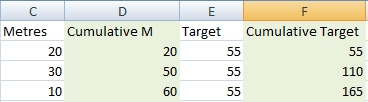# SQL show monh to date numbers

Hi

I use the following SQL statement to get the daily drill totals
Sum([Performance].[Drilled Total]) AS Metres
I now have to get the month to date totals based on the [Start Date]

``````SELECT [Performance].[Date] As [Date],[Performance].[Shaft], Sum([Performance].[Drilled Total]) AS Metres,[vShafts_StartDate].[Target] * Count(DISTINCT [Performance].[Date]) As Target, Avg([Performance].[Drilled Total]) As [Ave M/Mach/Shift], Sum([Performance].[Delay Hours 1]) AS Delays, Sum([Performance].[DWR Hours]) AS DWR, Sum([Performance].[Standing Time]) AS [Standing Time], Sum([Performance].[Grout Hours]) AS [Grout Hours], Sum([Performance].[Concrete Redrill]) AS ReDrill, Sum([Performance].Setup) AS Setups, Sum([Performance].[Transport Hours]) as [Transport Hours], Sum([Performance].[Photographic Survey]) as [Photographic Survey], Sum([Performance].[Day Rate]) as [Day Rate], Sum([Performance].[Blank Cap]) as [Blank Cap], Sum([Performance].[Mobilise]) as [Mobilise], Sum([Performance].[Set Up Stope]) as [Set Up Stope], Sum([Performance].[Interhole Move]) as [Interhole Move], Sum([Performance].[Grouting Borehole]) as [Grouting Borehole], Sum([Performance].[Plugging]) as [Plugging], Sum([Performance].[Trp Equip UG]) as [Trp Equip UG], Cast([vShafts_StartDate].[StartDate] As Date) As [Month_Start], [vShafts_StartDate].[Target] as newTarget
FROM [Performance] Inner Join [vShafts_StartDate] On [Performance].[Shaft] = [vShafts_StartDate].[Shaft]
Where [Date] >= [vShafts_StartDate].[StartDate] And [Date] <= '11 Mar 2016'And [Performance].[Shaft] = 'kopanang air'
GROUP BY [Performance].[Date],[Performance].Shaft,[vShafts_StartDate].[StartDate],[vShafts_StartDate].[Target]
``````
Microsoft SQL ServerLast Comment
Murray BrownBrian Crowe``````SELECT [Performance].[Date] As [Date],
[Performance].[Shaft],
SUM([Performance].[Drilled Total]) AS Metres,
[vShafts_StartDate].[Target] * Count(DISTINCT [Performance].[Date]) As Target,
AVG([Performance].[Drilled Total]) As [Ave M/Mach/Shift],
SUM([Performance].[Delay Hours 1]) AS Delays,
SUM([Performance].[DWR Hours]) AS DWR,
SUM([Performance].[Standing Time]) AS [Standing Time],
SUM([Performance].[Grout Hours]) AS [Grout Hours],
SUM([Performance].[Concrete Redrill]) AS ReDrill,
SUM([Performance].Setup) AS Setups,
SUM([Performance].[Transport Hours]) as [Transport Hours],
SUM([Performance].[Photographic Survey]) as [Photographic Survey],
SUM([Performance].[Day Rate]) as [Day Rate],
SUM([Performance].[Blank Cap]) as [Blank Cap],
SUM([Performance].[Mobilise]) as [Mobilise],
SUM([Performance].[Set Up Stope]) as [Set Up Stope],
SUM([Performance].[Interhole Move]) as [Interhole Move],
SUM([Performance].[Grouting Borehole]) as [Grouting Borehole],
SUM([Performance].[Plugging]) as [Plugging],
SUM([Performance].[Trp Equip UG]) as [Trp Equip UG],
CAST([vShafts_StartDate].[StartDate] As Date) As [Month_Start],
[vShafts_StartDate].[Target] as newTarget,
SUM(CASE WHEN YEAR([Performance].[Date]) = YEAR(GETDATE()) AND MONTH([Performance].[Date]) = MONTH(GETDATE()) THEN [Performance].[Drilled Total] ELSE 0 END) AS MTDDrillTodal
FROM [Performance]
INNER JOIN [vShafts_StartDate]
ON [Performance].[Shaft] = [vShafts_StartDate].[Shaft]
WHERE [Performance].[Date] >= [vShafts_StartDate].[StartDate]
AND [Performance].[Date] <= CAST(GETDATE() AS DATE)
AND [Performance].[Shaft] = 'kopanang air'
GROUP BY [Performance].[Date], [Performance].Shaft, [vShafts_StartDate].[StartDate], [vShafts_StartDate].[Target]
``````Jim HornJust to make sure we're not missing anything, give us a data mockup of both your current data and your desired return set.Murray BrownHere is an example of the  two columns that I want to get a cumulative total on. I have also attached a spreadsheet showing what all columns would look likeBook2.xlsxScott PletcherTHIS SOLUTION IS ONLY AVAILABLE TO MEMBERS.
View this solution by signing up for a free trial.
Members can start a 7-Day free trial and enjoy unlimited access to the platform.Murray BrownThanks very muchMicrosoft SQL Server

Microsoft SQL Server is a suite of relational database management system (RDBMS) products providing multi-user database access functionality.SQL Server is available in multiple versions, typically identified by release year, and versions are subdivided into editions to distinguish between product functionality. Component services include integration (SSIS), reporting (SSRS), analysis (SSAS), data quality, master data, T-SQL and performance tuning.

171K
Questions
--
Followers
--
Top Experts
Get a personalized solution from industry experts

TRUSTED BY